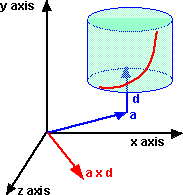# Maths - Dual Vectors

## Prerequisites

If you are not familiar with this subject you may like to look at the following pages first:

## Description

Dual numbers have the form:

a + w b

where:

w2 = 0

In the case where w2 = 0 we indicate this by replacing w with ε to give:

a + ε b

for dual vectors 'a' and 'b' can be vectors.

This is similar to the way that quaternions can be represented as a real part (scalar) and an imaginary part which is a vector.

For dual vectors either or both parts may be vectors.

### Relationship to 3D Geometric/Clifford algebra

What is the relationship between dual vectors and 3D Geometric/Clifford algebra?

dual vectors has:

• two 3D vectors

3D Geometric/Clifford algebra has:

• two 3D vectors and two scalars

So could 3D Geometric algebra be a superset of dual vectors?

In 3D Geometric algebra squaring either of the vectors will give a scalar value, so if we dont have a scalar value then perhaps squaring them will give zero?

### Applications of dual vectors

Dual vectors can be used to represent 'screw displacement' that is, a representation of velocity of a solid body, a combination of linear and angular velocity. (see kinimatics page on this subject).The components of a and d are known as plucker coordinates.

To represent this using the dual vector a + w b

then b = a x d

where:

• a is the distance from origin to axis vector.
• b is the w part of dual vector.
• d is the direction of the axis vector.
• x is the cross product